Solving More Decimal Word Problems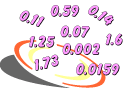Example 1: School lunches cost \$14.50 per week. About how much would 15.5 weeks of lunches cost?

Analysis: We need to estimate the product of \$14.50 and 15.5. To do this, we will round one factor up and one factor down.

Estimate: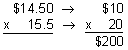Answer: The cost of 15.5 weeks of school lunches would be about \$200.Example 2: A student earns \$11.75 per hour for gardening. If she worked 21 hours this month, then how much did she earn?

Analysis: To solve this problem, we will multiply \$11.75 by 21.

Multiply: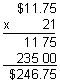Answer: The student will earn \$246.75 for gardening this month.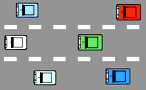Example 3: Rick's car gets 29.7 miles per gallon on the highway. If his fuel tank holds 10.45 gallons, then how far can he travel on one full tank of gas?

Analysis: To solve this problem, we will multiply 29.7 by 10.45

Multiply: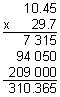Answer: Rick can travel 310.365 miles with one full tank of gas.Example 4: A member of the school track team ran for a total of 179.3 miles in practice over 61.5 days. About how many miles did he average per day?

Analysis: We need to estimate the quotient of 179.3 and 61.5.

Estimate: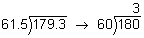Example 5: A store owner has 7.11 lbs. of candy. If she puts the candy into 9 jars, how much candy will each jar contain?

Analysis: We will divide 7.11 lbs. by 9 to solve this problem.

Divide: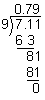Answer: Each jar will contain 0.79 lbs. of candy.Example 6: Paul will pay for his new car in 36 monthly payments. If his car loan is for \$19,061, then how much will Paul pay each month? Round your answer to nearest cent.

Analysis: To solve this problem, we will divide \$19,061.00 by 36, then round the quotient to the nearest cent (hundredth).

Divide:Answer: Paul will make 36 monthly payments of \$529.47 each.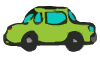Example 7: What is the average speed in miles per hour of a car that travels 956.4 miles in 15.9 hours? Round your answer to the nearest tenth.

Analysis: We will divide 956.4 by 15.9, then round the quotient to the nearest tenth.

Step 1: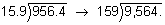Step 2:Answer: Rounded to the nearest tenth, the average speed of the car is 60.2 miles per hour.

Summary: In this lesson we learned how to solve word problems involving decimals. We used the following skills to solve these problems:

1. Estimating decimal products
2. Multiplying decimals by whole numbers
3. Multiplying decimals by decimals
4. Estimating decimal quotients
5. Dividing decimals by whole numbers
6. Rounding decimal quotients
7. Dividing decimals by decimals

Exercises

Directions: Read each question below. You may use paper and pencil to help you solve these problems. Click once in an ANSWER BOX and type in your answer; then click ENTER. After you click ENTER, a message will appear in the RESULTS BOX to indicate whether your answer is correct or incorrect. To start over, click CLEAR.

 1.Estimate the amount of money you need to pay for a tank of gas if one gallon costs \$3.04 and the tank holds 11.9 gallons. ANSWER BOX: \$  RESULTS BOX:
 2.The sticker on Dean's new car states that the car averages 32.6 miles per gallon. If the fuel tank holds 12.3 gallons, then how far can Dean travel on one full tank of gas? ANSWER BOX: miles   RESULTS BOX:
 3.Larry worked 15 days for a total of 116.25 hours. How many hours did he average per day? ANSWER BOX: hours  RESULTS BOX:
 4.Six cases of paper cost \$159.98. How much does one case cost? Round your answer to the nearest cent.  ANSWER BOX: \$  RESULTS BOX:
 5.There are 2.54 centimeters in one inch. How many inches are there in 51.78 centimeters? Round your answer to the nearest thousandth. ANSWER BOX:  in.   RESULTS BOX: